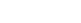# SAT Math Basics – Triangles

What you need to know about triangles on the SAT is nowhere near as much as you probably learned in high school. Remember law of cosines? Exactly, most people do not. But the law of cosines, and just about every thing else from trigonometry, is not tested on the SAT.

Ahh…I think I just heard an audible collective sigh of relief. While the SAT only tests basics aspects of triangles, it will try to trick you whenever possible. Know the 10 tips below and you should be fine.

1. The degrees of a triangle add up to 180

2. An isosceles triangle has exactly two equal sides

3. An equilateral triangle has three equal sides. Each angle of the triangle is equal to 60

4. A right triangle has one side that equals 90 degrees.

5. An isosceles right triangle is a right triangle in which the two shorter sides of a triangle are equal to one another. Each of the other two angles have a measure of 45 degrees.

6. The greater the degree measure of an angle, the greater the length of the side opposite from that angle.

7. The longest side of a triangle is called the hypotenuse.

8. The sum of the square of the lengths of the two shorter sides of a right triangle equals the square of the hypotenuse:, where a and b are the two shorter sides of a right triangle.

9. The base and the height of a triangle must always meet at 90 degrees.

10. The area of a triangle is base x height divided by 2.

## Takeaway

But with a little practice, and by memorizing and applying the 10 tips above, you are well on your way to triangle mastery on the SAT.

## Author

•Chris Lele is the Principal Curriculum Manager (and vocabulary wizard) at Magoosh. Chris graduated from UCLA with a BA in Psychology and has 20 years of experience in the test prep industry. He's been quoted as a subject expert in many publications, including US News, GMAC, and Business Because. In his time at Magoosh, Chris has taught countless students how to tackle the GRE, GMAT, SAT, ACT, MCAT (CARS), and LSAT exams with confidence. Some of his students have even gone on to get near-perfect scores. You can find Chris on YouTube, LinkedIn, Twitter and Facebook!